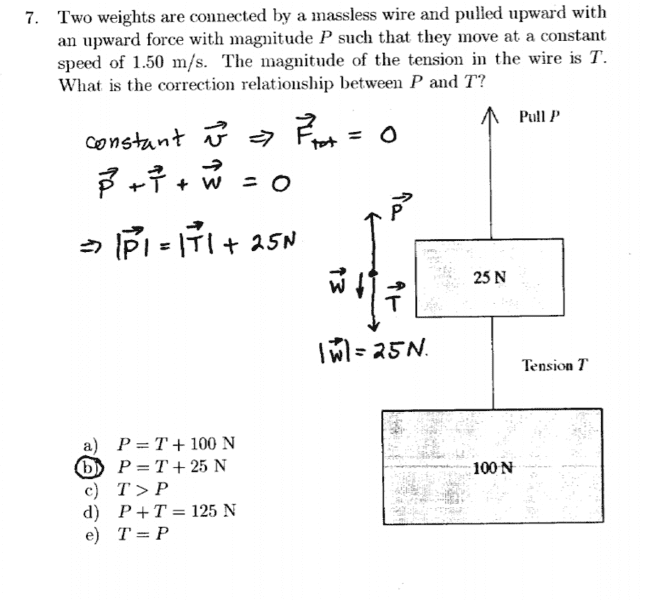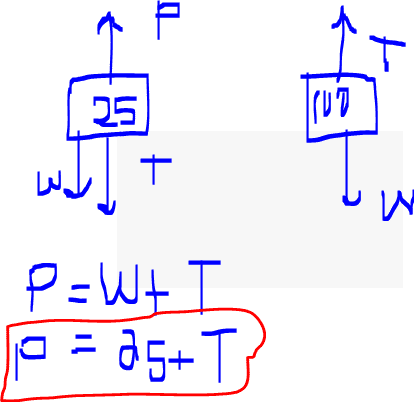# Tension In a String

## Homework Statement## Homework Equations

Ook, so i'm really bad at physics, and i need someone to help explain why the answer is P=T+25 N
I thought it is P+T = 125

## The Attempt at a Solution

Since the system is in constant velocity, then Fnet = 0 so that P + T + W = 0 Why isn't it P + T + 125 =0?[/B]

## Answers and Replies

Homework Helper
Which direction does T pull on the 100 N weight?
Which direction does T pull on the 25 N weight?

Which direction does T pull on the 100 N weight?
Which direction does T pull on the 25 N weight?
T always pull away from the object. So, for the 100 N weight, Tension pulls downward and for 25N, the tension pulls upward

Homework Helper
Gold Member
T always pull away from the object.
Yes.
So, for the 100 N weight, Tension pulls downward ...
Away from the 100N weight would be upwards, surely?

Homework Helper
Gold Member
Perhaps draw a two free body diagrams, one for each mass.

•Lori
Perhaps draw a two free body diagrams, one for each mass.
Thanks , i drew it for the 25 N object and got that P = T+25!#### Attachments

Last edited by a moderator:
•jbriggs444
I have a question to add!
If i know that W = T for the second diagram , then I know that T = 100N right?

I can actually find P since P = 25+T , so that P=125

•CWatters
•